# Chapter 3 Graphing Linear Equations Section 4 Linear

• Slides: 12Chapter 3 Graphing Linear Equations Section 4 Linear Functions Copyright © 2016, 2012, and 2009 Pearson Education, Inc. 1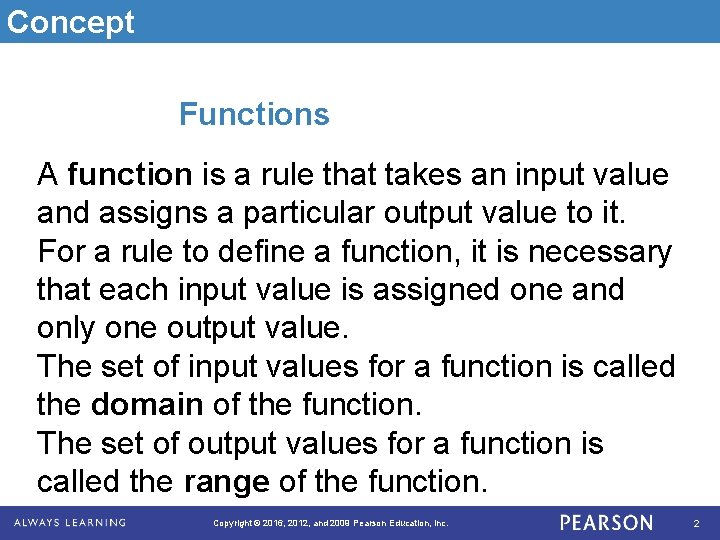Concept Functions A function is a rule that takes an input value and assigns a particular output value to it. For a rule to define a function, it is necessary that each input value is assigned one and only one output value. The set of input values for a function is called the domain of the function. The set of output values for a function is called the range of the function. Copyright © 2016, 2012, and 2009 Pearson Education, Inc. 2Example A health club charges a \$75 initiation fee plus \$29 per month. Find the function for the total cost of joining the health club, as well as the domain and range of the function. Solution Function If n is the number of months, then the cost is equal to 29 n + 75. Domain: Set of possible months {1, 2, 3, 4, …} Range: Set of possible costs {\$104, \$133, \$162, …} Copyright © 2016, 2012, and 2009 Pearson Education, Inc. 3Example Functions Is a relation whose input value is a student at your college and whose output is the student’s ID number a function? Yes, because each student has only one ID number. Copyright © 2016, 2012, and 2009 Pearson Education, Inc. 4Concept Function Notation Function notation is a way to present the formula for the output value of a function for the input x. Copyright © 2016, 2012, and 2009 Pearson Education, Inc. 5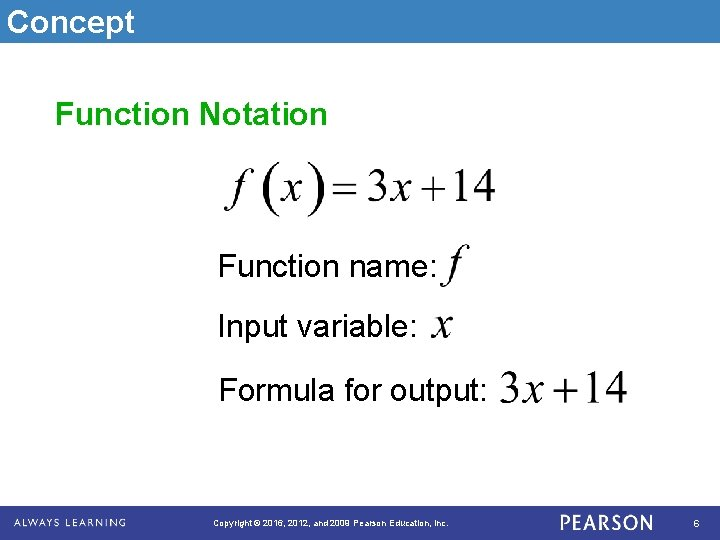Concept Function Notation Function name: Input variable: Formula for output: Copyright © 2016, 2012, and 2009 Pearson Education, Inc. 6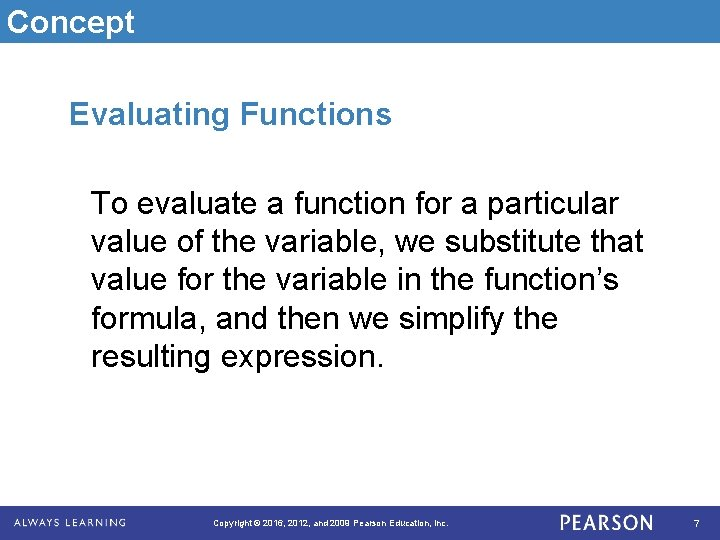Concept Evaluating Functions To evaluate a function for a particular value of the variable, we substitute that value for the variable in the function’s formula, and then we simplify the resulting expression. Copyright © 2016, 2012, and 2009 Pearson Education, Inc. 7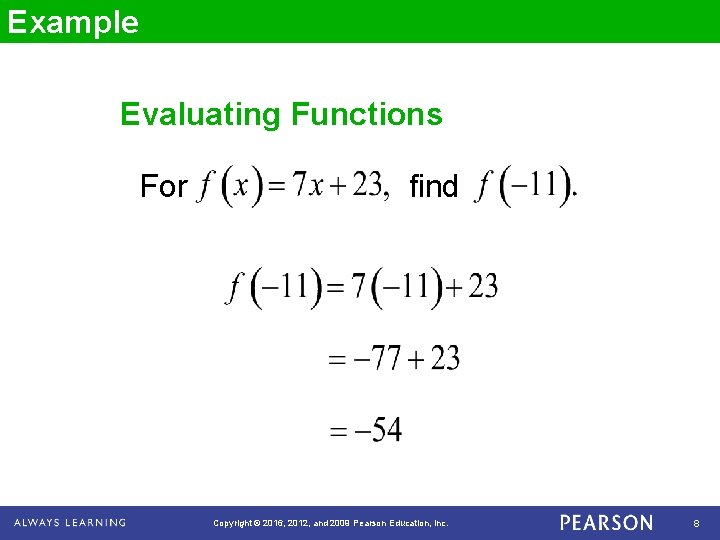Example Evaluating Functions For find Copyright © 2016, 2012, and 2009 Pearson Education, Inc. 8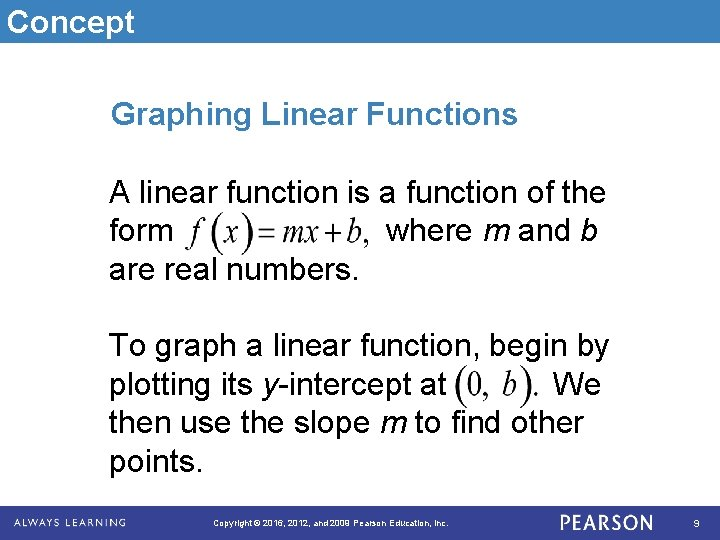Concept Graphing Linear Functions A linear function is a function of the form where m and b are real numbers. To graph a linear function, begin by plotting its y-intercept at We then use the slope m to find other points. Copyright © 2016, 2012, and 2009 Pearson Education, Inc. 9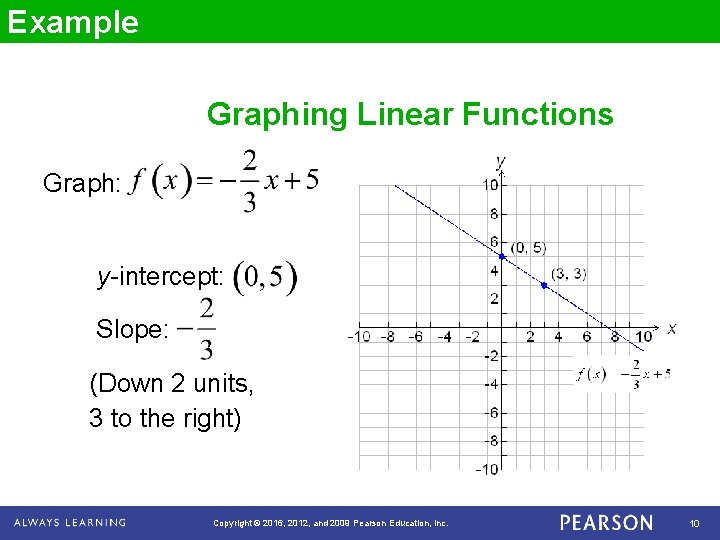Example Graphing Linear Functions Graph: y-intercept: Slope: (Down 2 units, 3 to the right) Copyright © 2016, 2012, and 2009 Pearson Education, Inc. 10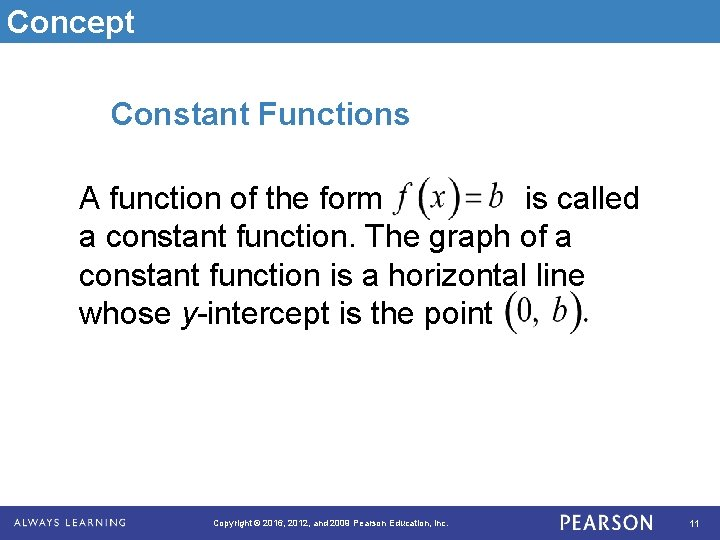Concept Constant Functions A function of the form is called a constant function. The graph of a constant function is a horizontal line whose y-intercept is the point Copyright © 2016, 2012, and 2009 Pearson Education, Inc. 11Example Constant Functions Graph: Copyright © 2016, 2012, and 2009 Pearson Education, Inc. 12